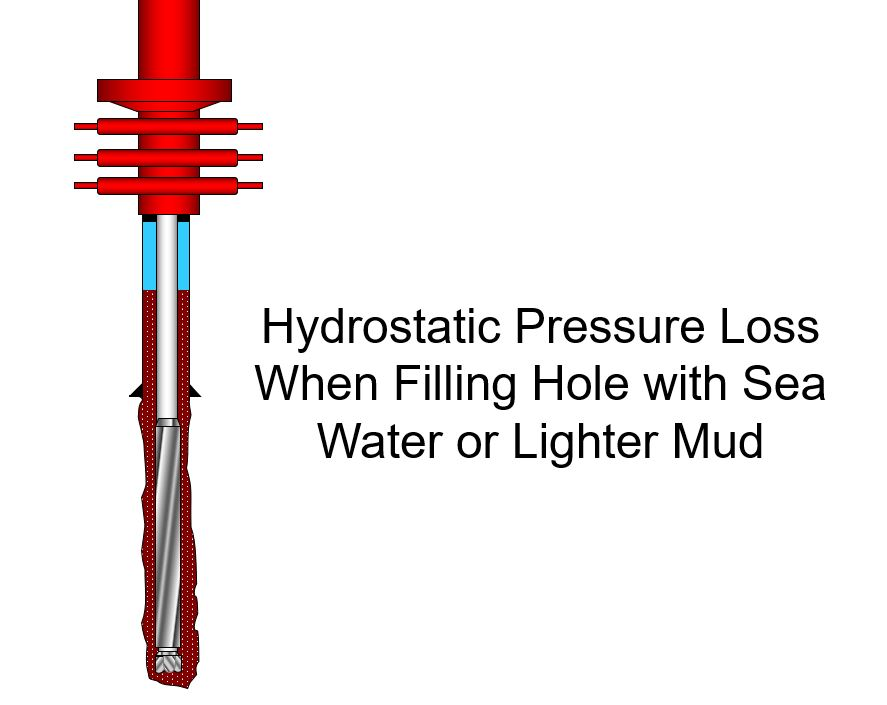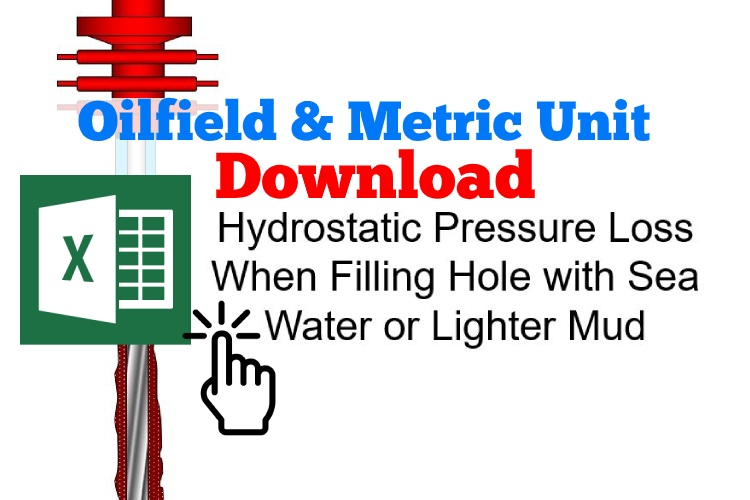# Loss of Hydrostatic Pressure When Filling Hole with Water or Lighter Mud

In case of totally lost return, the annulus must be fully filled with fluid, normally water, as fast as we can. Water filled in annulus causes loss of hydrostatic pressure in the wellbore. This article demonstrates how to determine hydrostatic pressure reduction when water or other light fluid is used to fully fill the hole.Note: This calculation is based on if the float is in the drill string. Therefore, there is no way that fluid from annulus can come into the string.

There are two main concepts, annular capacity and hydrostatic pressure, applied to determine loss of hydrostatic pressure.

## Oilfield Unit

Height of feet of water in annulus

Height of water added  (ft)= water added in bbl ÷ annular capacity in bbl/ft

Bottomhole (BHP) pressure reduction

BHP decrease in psi = (current mud weight in ppg – weight of water in ppg) × 0.052 × (ft of water added)

Note: In order to calculate bottom hole pressure reduction, we assume the column of water in annulus is true vertical depth (TVD). This calculation may not be accurate if the well has high angle so you need to determine the actual TVD from directional survey data. However, this method will be the worst case scenario of pressure reduction.

Equivalent Mud Weight at TD

EMW in ppg = current mud weight in ppg – (BHP decrease in psi ÷ 0.052 ÷ TVD ft of hole)

Example: Determine bottom hole pressure loss and equivalent mud weight at TD due to filling up water into annulus.

Mud weight = 13.0 ppg
Water added = 140 bbl required to fill annulus
Weight of water = 8.6 ppg **
Annular capacity = 0.1422 bbl/ft
Hole TVD = 6,000 ft

Number of feet of water in annulus

Feet of water in annulus = 140 bbl ÷ 0.1422 bbl/ft

Feet = 984.5 ft

Bottomhole (BHP) pressure reduction

BHP reduction = (13.0 ppg – 8.6 ppg) × 0.052 × 984.5 ft

BHP reduction = 225.3 psi

Equivalent mud weight at TD

EMW in ppg = 13.0 – (225.3 psi ÷ (0.052× 6,000 ft))

EMW = 12.3 ppg

## Metric Unit

Height of water in annulus

Height of water added  (m) = water added in m3 ÷ annular capacity in m3/m

Bottomhole (BHP) pressure reduction

BHP decrease in psi = (current mud weight in kg/m3 – weight of water in kg/m3) × 0.00981 × (m of water added)

Equivalent Mud Weight at TD

EMW in ppg = current mud weight in kg/m3 – (BHP decrease in KPa ÷ 0.00981 ÷ TVD m of hole)

Example: Determine bottom hole pressure loss and equivalent mud weight at TD due to filling up water into annulus.

Mud weight = 1550 kg/m3
Weight of water = 1030 kg/m3
Annular capacity = 0.07417 m/m
Hole TVD = 2000 m

Height of water in annulus

Height of water in annulus= 20 m3 ÷ 0.07417 m3/m

Height of water in annulus = 270 m

Bottomhole (BHP) Pressure Reduction

BHP reduction = (1550 kg/m3 – 1030 kg/m3) × 0.00981 × 270 m

BHP reduction = 1,375 KPa

Equivalent mud weight at TD

EMW   = 1550 – (1375 ÷ (0.00981× 2,000 m))

EMW = 1480 kg/m3

Please find the Excel sheet for calculating how much pressure loss due to lost returnRef books: Lapeyrouse, N.J., 2002. Formulas and calculations for drilling, production and workover, Boston: Gulf Professional publishing.

Bourgoyne, A.J.T., Chenevert , M.E. & Millheim, K.K., 1986. SPE Textbook Series, Volume 2: Applied Drilling Engineering, Society of Petroleum Engineers.

Mitchell, R.F., Miska, S. & Aadny, B.S., 2011. Fundamentals of drilling engineering, Richardson, TX: Society of Petroleum Engineers.

Share the joyWorking in the oil field and loving to share knowledge.

### 3 Responses to Loss of Hydrostatic Pressure When Filling Hole with Water or Lighter Mud

1.obes umi says:

sir. ilove ur drilling formulas and drilling calculations i need some of your example of all the drilling calculation formulas.
2.Kevin says:
•DrillingFormulas.Com says: